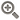﻿ ​债券到期收益率计算函数-点掌财经• 综合
• 股票
• 板块
• 嘉宾
• 课程
• 基金
• 经理
• 说说
• 掌评
• 消息
• 好看
• 话题

# ​债券到期收益率计算函数Public Function ins(pur As Double, par As Double, rate As Double, nper As Double) As Double

'四个变量，pur购买价值，par 票面价值，rate 必要报酬率（或折现率），nper复利期数

Dim i As Double, pur1 As Double, pur2 As Double

For i = 0.01 To 1 Step 0.01

pur1 = par * rate * (1 - (1 + i) ^ (-nper)) / i + par * (1 + i) ^ (-nper)

pur2 = par * rate * (1 - (1 + (i + 0.01)) ^ (-nper)) / (i + 0.01) + par * (1 + (i + 0.01)) ^ (-nper)

'分别用1到100的利率去试验债券的现值，直到找到一个利率，使债券的现值大于或等于债券的购买价值，而当利率再上升的时候，就会小于债券的购买价值

If pur <= pur1 And pur >= pur2 Then

ins = Round((pur - pur1) / (pur2 - pur1) * ((i + 0.01) - i) + i, 4)

'用内插法，将三个值近似当作直线计算，求出真正让债券的现值等于购买价值的利率

Exit For

End If

Next

'本函数仅局限于普通债券的到期收益率的计算。股票模型思路一样，但函数需稍有变动。

'实际题目或者考试中可能会给出等待试验的两至三个利率，并不需要像本函数中从1%至100%试验。实际上考试中给的利率的间隔也不一定是1%.（必要报酬率也不可能高达100%），但是内插法的基本思路一样。

'本例仅为题目未给出让你试验的利率的时候，大致估算债券的到期收益率。 End Function

ins(1000,1000,8%,5)=8%  到期收益率8%等于票面利率8%

ins(1000,1000,8%/2,5*2)=4%  一年内复利两次，即每半年付息。到期收益率也与票面利率一致(本例计算为4%，为半年的利率)

ins(1000,1000,8%,10)=8%  无论到期时间的长短，到期收益率均等于票面利率

ins(1100,1000,8%,5)=5.65%  到期收益率5.65%小于票面利率8%

ins(1100,1000,8%/2,5*2)=2.84%  即一年内复利两次，即每半年付息。到期收益率为2.84%*2=5.68%〉5.65%，印证了“溢价债券付息越频繁，债券价值越大”的结论

ins(1100,1000,8%,2)=2.79%  到期时间越短，到期收益率越小，与教材中的“溢价债券越接近到期日价值越小”结论一致

ins(900,1000,8%,5)=10.69%  到期收益率10.69%大于票面利率8%

ins(900,1000,8%/2,5*2)=5.33%  即一年内复利两次，即每半年付息。到期收益率为5.33%*2=10.66%《10.69%，印证了“折价债券付息越频繁，债券价值越小”的结论

ins(900,1000,8%,2)=14.08%  到期时间越短，到期收益率越大，与教材中的“折价债券越接近到期日价值越大”结论一致

#### 最新发布• 手机号码：

请输入手机号码，您的个人信息严格保密，请放心

• 图形验证码：

请输入右侧图形验证码

• 短信验证码：

请输入接收的短信验证码

获取短信验证码
X• 10牛
• 30牛
• 50牛
• 其它